• 符号位只是两者的异或，指数位基本上是指数的相加，而尾数位就需要在正常的乘法之余考虑到移位（和随之而来的指数为的溢出）和进位的情况。 下面就来讨论一下尾数的运算： 在尾数前补上1，进行无符号数乘法
按：计算机组成课程第四周作业
算法证明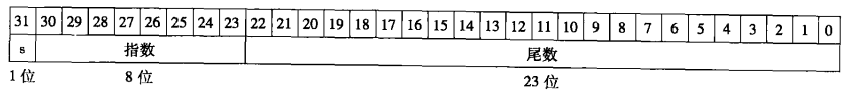图表 1       浮点数的表示
浮点数的表示如上图所示，我们要做的是将按如上方式存储的两个浮点数相乘，将其结果用如上的方式表示。
符号位只是两者的异或，指数位基本上是两指数的相加，而尾数位就需要在正常的乘法之余考虑到移位（和随之而来的指数为的溢出）和进位的情况。
下面就来讨论一下尾数的运算：
在尾数前补上1，进行无符号数乘法。小数点仅作为算法分析时的记号，实际上不参加运算。用64位的long long类型的数来存储运算结果。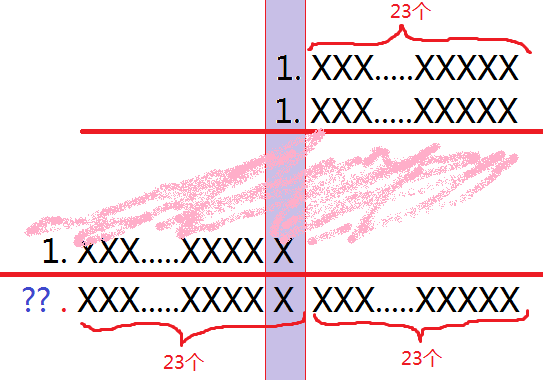图表 2       尾数相乘（用“画图”程序画的）
如上图所示，后46个数字是结果的小数点后的数据，小数点的的问号处可能只有1，可能是1X（10或11，在算法中没有太大区别）。若问号处只有1，说明已经规格化完成；若是1X，需要将整个数右移一位，质数加一，从而使问号处只有1。
规格化后要进行round操作，如下图所示。如果第23位是0，这一位之前的23位就是所需要的尾数。如果第23位是1，截取这位之前的数，加一（类似于四舍五入）。判断结果是否仍规格化。若没有规格化，从规格化开始继续做，直到找到规格化后的23位尾数。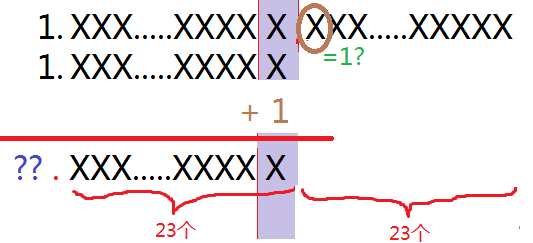图表 3       有效数处理（用“画图”程序画的）
程序框图
主要流程如下图所示。在代码注释中的Step1到step5即分别对应流程图中的1至5部分。有所不同的是，因为后面只会增加exponent而不会减少，所以我的程序在step1就判断是否overflow，在step3也判断是否overflow就行了，规格化完成后再判断underflow。图表 4       浮点乘法程序框图
使用方法
输入两个数，程序用自带的scanf将其作为单精度浮点数读入。通过一系列的无符号数的计算，得到用单精度浮点数表示的结果，将其输出（为了更详细地看到整个数，输出的数小数点后有20位）。如果overflow或underflow，输出的是相应的提示。为了验证计算是否正确，在输出的数据后面有个括号，里面放了是直接让机器进行的单精度浮点数的乘法的结果。
特殊处理
特殊输入数据
有些输入的数据是在太大或太小，以至于数据本身就已经overflow或underflow，从而根本无法存贮到单精度浮点数中，不是合法的单精度浮点数。对于这样的数，我们不应该对其提供任何服务，不然只会闹出笑话。
对于零要特殊处理，因为0在浮点数中的表示并不是按照一般的规范来的。
溢出
这里的溢出指的是乘数和被乘数本身是合法的单精度浮点数，但相乘后造成了溢出。
因为后面只会增加exponent而不会减少，所以与上面的流程图不同，我的程序在step1就判断是否overflow，在step3也判断是否overflow就行了，规格化完成后再判断underflow。
数位扩展
整个程序都是按照单精度的浮点数来写的，数位扩展可能有点麻烦。如果要扩展为双精度的，需要更改代码中的大量参数。鉴于浮点数一般也就只有单精度和双精度两种，我觉得不是太必要提高做成可扩展的。
实例分析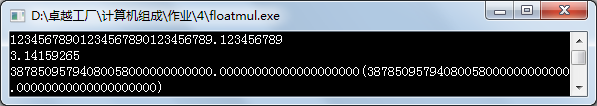图表 5       大数的乘法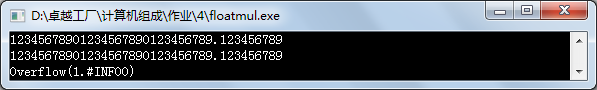图表 6       overflow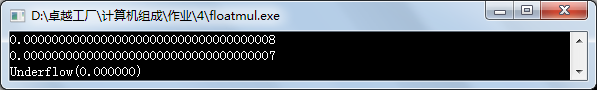图表 7       underflow（本程序判为underflow，而C语言自己的浮点数乘法计算结果为0.000000）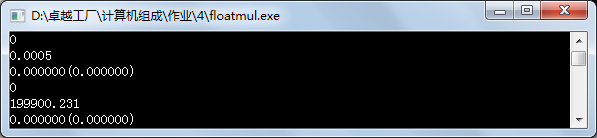图表 8       对0的乘法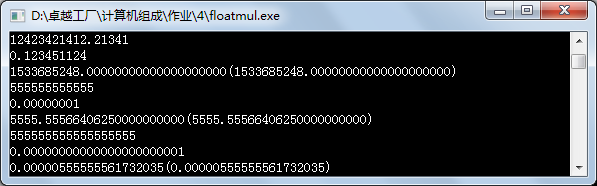图表 9       很大的与很小的正数相乘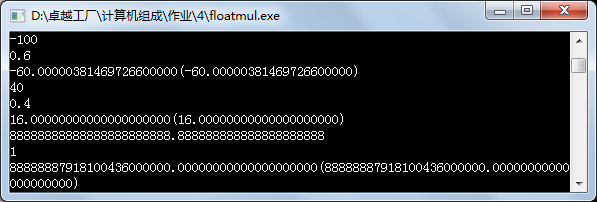图表 10     观察精确度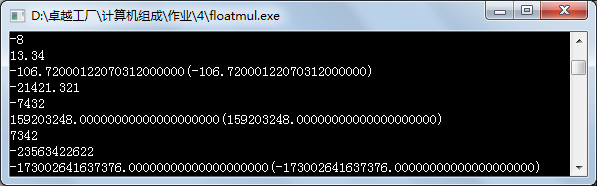图表 11     对负数乘法的测试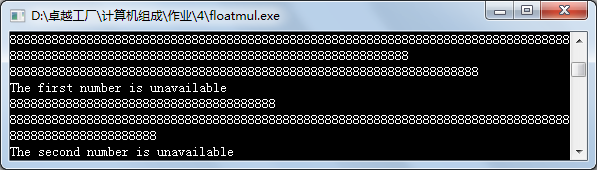图表 12     对于无法用浮点数表示的输入数据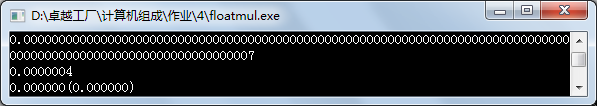图表 13     对于过小的数据
结果分析
我们对过于大的数据和过于小的数据，以及精度太大的数据都做了试验。从上述结果可以看到，这个程序的结果与C程序自带的浮点数乘法结果基本吻合。说明这个程序还是比较可靠的。
通过对精度的测试，我们发现浮点数虽然能表示较大较小的数据，但有效数字还是有限的。如-100与0.6相乘的结果，整数部分60是对的，小数点五位后就出现了偏差。一连串8与1相乘，结果的头部只有7个8。可以大致地认为浮点数的表示与计算的十进制有效数位是7位。
可以更进一步的工作
l  程序中对于无符号整数的加法、乘法，对于exponent的有符号整数运算都是直接使用的，其实可以调用之前写的相关程序，做成更加本质的浮点数乘法。
Source Code

#include<stdio.h>
union unionfloat{
float f;
unsigned long l;
};

union unionfloat x,y,product;
/*
disp(union unionfloat x)
{
long i;
unsigned long m;
printf("%f:",x.f);
m=0x80000000;
for(i=0; i<32; i++){
if((i&7)==0)printf(" ");
if(m&x.l)printf("1");
else printf("0");
m>>=1;
}
printf("\n");
}*/
int mul(void){
//Step 1
int exponent;
exponent=(x.l&0x7F800000)>>23;
exponent-=0x7F;
exponent+=(y.l&0x7F800000)>>23;
exponent-=0x7F;
if(exponent>=128) return 1;

//Step 2
unsigned long long raw_product;
unsigned long long raw_x=x.l&0x007FFFFF|0x00800000;
unsigned long long raw_y=y.l&0x007FFFFF|0x00800000;
int carry;
raw_product = raw_x * raw_y;

/*	int i=64;
unsigned long long p=0x8000000000000000;
while(i--){
if(i%4==3)printf(" ");
if(raw_product&p)printf("1");
else printf("0");
p=p>>1;
}
printf("\n");*/

//相乘结果若小数点前有两位
do{
//Step 3
if(raw_product>>47){
raw_product = raw_product>>1;
exponent++;
if(exponent>=128) return 1;//printf("Overflow");
}

//Step 4
if(raw_product & 0x0000000000400000){
raw_product = ((raw_product>>23)+1)<<23;
}

}while(raw_product>>47);//still normalized?

//Step 5
product.l = ((x.l>>31)^(y.l>>31))<<31;
if(exponent<=-127)	return -1;//printf("Underflow");
//-127<exponent<128
product.l = product.l | ((unsigned long)(exponent+127))<<23;
product.l = product.l|((unsigned long)((raw_product&0x00003FFFFF800000)>>23));
//disp(product);
printf("%.20f(%.20f)\n",product.f,x.f*y.f);
return 0;
}

int main(void){

while(~scanf("%f%f",&x.f,&y.f)){
//printf("Input %.10f %.10f\n",x.f,y.f);
//disp(x);disp(y);
if(x.l==0||y.l==0)
printf("%f(%f)\n",0,x.f*y.f);
else if((((x.l>>23)&0x000000FF)==0) || (((x.l>>23)&0x000000FF)==0x000000FF))
printf("The first number is unavailable\n");
else if((((y.l>>23)&0x000000FF)==0) || (((y.l>>23)&0x000000FF)==0x000000FF))
printf("The second number is unavailable\n");
else{
switch(mul()){
case 1:printf("Overflow(%f)\n",x.f*y.f);break;
case -1:printf("Underflow(%f)\n",x.f*y.f);break;
default:break;
}
}
}
return 0;
}

展开全文c语言 算法
• 在一些诸如特征比对等等的应用场景中，特征值其实就是一个个的浮点数(float)组成的矩阵，这些浮点数每一个都是4个字节(32位），然后对这个矩阵进行乘法计算，A * B = C，得出的C矩阵就是能够代表个特征矩阵相似...
        在一些诸如特征比对等等的应用场景中，特征值其实就是一个个的浮点数(float)组成的矩阵，这些浮点数每一个都是4个字节(32位），然后对这两个矩阵进行乘法计算，A * B = C，得出的C矩阵就是能够代表两个特征矩阵相似性的值。毫无疑问矩阵乘法可以在CPU中进行计算，但是，在一些需要实时性的场合下，CPU的运算速度可能就不能满足要求，而通过GPU去做矩阵乘法反而再合适不过，GPU处理浮点数运算的能力非常强，这里记录两种接口来使用GPU计算矩阵乘法。

首先，两个矩阵相乘有意义必须满足：矩阵A为m行n列，矩阵B为n行k列，则矩阵C=AB即C为m行k列。使用cuda的runtime库和cublas库在GPU中进行计算。现在CPU中使用随机浮点数产生两个矩阵h_A和h_B，再使用cublasSetVector函数或cudaMemcpy函数把h_A和h_B从CPU内存中复制到GPU中相应的d_A和d_B中，如果是float型，可以直接使用cublasSegmm函数进行矩阵乘法运算，结果置于d_C中，若为int8型，则可使用cublasGemmEx函数，再使用cublasGetVector函数或cudaMemcpy函数将d_C从GPU内存中复制到CPU内存中。

cublasSegmm函数做FP32型矩阵乘法实现：

   A:M行N列矩阵  B:N行M列矩阵  C:M行M列矩阵

#include "cuda_runtime.h"
#include "cublas_v2.h"
#include <iostream>

using namespace std;

#define M 5
#define N 3

int main()
{
// 定义状态变量，矩阵乘法接口的返回值
cublasStatus_t status;

// 在 CPU内存 中为将要计算的矩阵开辟空间
float *h_A = (float*)malloc (N*M*sizeof(float));
float *h_B = (float*)malloc (N*M*sizeof(float));

// 在 CPU内存 中为将要存放运算结果的矩阵开辟空间
float *h_C = (float*)malloc (M*M*sizeof(float));

// 为待运算矩阵的元素赋予 0-10 范围内的随机数，实际使用时，A矩阵需要做转置，B矩阵不需要转      置，可以手动转置也可以算法内转置
for (int i=0; i<N*M; i++)
{
h_A[i] = (float)(rand()%10+1);
h_B[i] = (float)(rand()%10+1);
}

// 打印待测试的矩阵
cout << "矩阵 A :" << endl;
for (int i = 0; i < N * M; i++){
cout << h_A[i] << " ";
if ((i + 1) % N == 0)
cout << endl;
}
cout << endl;
cout << "矩阵 B :" << endl;
for (int i = 0; i < N * M; i++){
cout << h_B[i] << " ";
if ((i + 1) % M == 0)
cout << endl;
}
cout << endl;

/*
** GPU 计算矩阵相乘
*/

// 创建并初始化 CUBLAS 库对象
cublasHandle_t handle;
status = cublasCreate(&handle);

if (status != CUBLAS_STATUS_SUCCESS)
{
if (status == CUBLAS_STATUS_NOT_INITIALIZED) {
cout << "CUBLAS 对象实例化出错" << endl;
}
getchar ();
return EXIT_FAILURE;
}

float *d_A, *d_B, *d_C;
// 在 显存 中为将要计算的矩阵开辟空间
cudaMalloc (
(void**)&d_A,    // 指向开辟的空间的指针
N*M * sizeof(float)    //　需要开辟空间的字节数
);
cudaMalloc (
(void**)&d_B,
N*M * sizeof(float)
);

// 在 显存 中为将要存放运算结果的矩阵开辟空间
cudaMalloc (
(void**)&d_C,
M*M * sizeof(float)
);

// 将矩阵数据传递进 显存 中已经开辟好了的空间
cublasSetVector (
N*M,    // 要存入显存的元素个数
sizeof(float),    // 每个元素大小
h_A,    // 主机端起始地址
1,    // 连续元素之间的存储间隔
d_A,    // GPU 端起始地址
1    // 连续元素之间的存储间隔
);
//注意：当矩阵过大时，使用cudaMemcpy是更好地选择：
//cudaMemcpy(d_A, h_A, sizeof(float)*N*M, cudaMemcpyHostToDevice);

cublasSetVector (
N*M,
sizeof(float),
h_B,
1,
d_B,
1
);
//cudaMemcpy(d_B, h_B, sizeof(float)*N*M, cudaMemcpyHostToDevice);
// 同步函数

// 传递进矩阵相乘函数中的参数，具体含义请参考函数手册。
float a = 1;
float b = 0;
// 矩阵相乘。该函数必然将数组解析成列优先数组
cublasSgemm (
handle,    // blas 库对象
CUBLAS_OP_T,    // 矩阵 A 属性参数
CUBLAS_OP_T,    // 矩阵 B 属性参数
M,    // A, C 的行数
M,    // B, C 的列数
N,    // A 的列数和 B 的行数
&a,    // 运算式的 α 值
d_A,    // A 在显存中的地址
N,    // lda
d_B,    // B 在显存中的地址
M,    // ldb
&b,    // 运算式的 β 值
d_C,    // C 在显存中的地址(结果矩阵)
M    // ldc
);

// 同步函数

// 从 显存 中取出运算结果至 内存中去
cublasGetVector (
M*M,    //  要取出元素的个数
sizeof(float),    // 每个元素大小
d_C,    // GPU 端起始地址
1,    // 连续元素之间的存储间隔
h_C,    // 主机端起始地址
1    // 连续元素之间的存储间隔
);

//或使用cudaMemcpy(h_C, d_C, sizeof(float)*M*M, cudaMemcpyDeviceToHost);
// 打印运算结果
cout << "计算结果的转置 ( (A*B)的转置 )：" << endl;

for (int i=0;i<M*M; i++)
{
cout << h_C[i] << " ";
if ((i+1)%M == 0) cout << endl;
}

// 清理掉使用过的内存
free (h_A);
free (h_B);
free (h_C);

try
{
cudaFree (d_A);
cudaFree (d_B);
cudaFree (d_C);
}
catch(...)
{
cout << "cudaFree Error!" << endl;
// 释放 CUBLAS 库对象
}

cublasDestroy (handle);
return 0;
}

但是GPU的内存一般比较有限，实际项目中，为了能够做更大的矩阵乘法，使用FP32型数据占用空间会很大，所以可以压缩为int8型数据进行矩阵乘法运算，这时使用的接口模型为：

cublasStatus_t cublasGemmEx(cublasHandle_t handle,      //句柄
cublasOperation_t transa,   //a矩阵转置选项
cublasOperation_t transb,   //b矩阵转置选项
int m,                      //a矩阵行数
int n,                      //b矩阵列数
int k,                      //a矩阵列数兼b矩阵行数
const void *alpha,          //乘法因子alpha
const void *A,              //A矩阵
int lda,                    //A矩阵的列数
const void *B,              //B矩阵
int ldb,                    //B矩阵的行数
const void *beta,           //乘法因子beta
void *C,                    //C结果矩阵
int ldc,                    //C矩阵的行数
cublasGemmAlgo_t algo)      

C=αop(A)op(B)+βC

其中，α和β为标量，A、B、C分别是m×k、k×n、m×n的矩阵，当α = 1, β = 0时，即为：

C=op(A)op(B)

也就是做矩阵乘法。α和β分别对应cublasGemmEx中的const void *alpha、const void *beta，这一点与前面cublasSegmm做FP32类型的接口是一样的。

在接口中，cublasOperation_t transa矩阵转置选项有如下三种：

transa == CUBLAS_OP_N表示A矩阵，无任何转置。

transa == CUBLAS_OP_T表示A^T，A的转置。

transa == CUBLAS_OP_C表示A^H，A的共轭转置。

同理，B矩阵也是一样。

computeType
Atype/Btype
Ctype

CUDA_R_16F

CUDA_R_16F

CUDA_R_16F

CUDA_R_32I

CUDA_R_8I

CUDA_R_32I

CUDA_R_32F

CUDA_R_16F

CUDA_R_16F

CUDA_R_8I

CUDA_R_32F

CUDA_R_16F

CUDA_R_32F

CUDA_R_32F

CUDA_R_32F

CUDA_R_64F

CUDA_R_64F

CUDA_R_64F

CUDA_C_32F

CUDA_C_8I

CUDA_C_32F

CUDA_C_32F

CUDA_C_32F

CUDA_C_64F

CUDA_C_64F

CUDA_C_64F

我们这里选择的int8型矩阵乘法，最好选择Atype/Btype为CUDA_R_8I型，计算模式CUDA_R_32I，乘出的结果Ctype为CUDA_R_32I类型。最后的cublasGemmAlgo_t参数可以选择的值如下表所示：

CublasGemmAlgo_t
含义

CUBLAS_GEMM_DEFAULT

Apply Heuristics to select the GEMM algorithm

CUBLAS_GEMM_ALGO0 to CUBLAS_GEMM_ALGO23

Explicitly choose an algorithm

CUBLAS_GEMM_DEFAULT_TENSOR_OP

Apply Heuristics to select the GEMM algorithm while allowing the use of Tensor Core operations if possible

CUBLAS_GEMM_ALGO0_TENSOR_OP to CUBLAS_GEMM_ALGO15_TENSOR_OP

Explicitly choose a GEMM algorithm allowing it to use Tensor Core operations if possible, otherwise falls back to cublas<t>gemmBatched based on computeType

这里可以选择CUBLAS_GEMM_ALGO0~7。

cublasGemmEX函数做int8型矩阵乘法实现：

#include "cuda_runtime.h"
#include "cublas_v2.h"
#include <iostream>
#include <math.h>

using namespace std;

#define M 5
#define N 3
#define BYTE 128

int main()
{
// 定义状态变量，矩阵乘法接口的返回值
cublasStatus_t status;

// 在 CPU内存 中为将要计算的矩阵开辟空间
char *h_A = (char *)malloc (N * M * sizeof(char));
char *h_B = (char *)malloc (N * M * sizeof(char));

// 在 CPU内存 中为将要存放运算结果的矩阵开辟空间
int *h_C = (int *)malloc ( M * M * sizeof(int));

float *f_A = (float *)malloc(N * M * sizeof(float));
float *f_B = (float *)malloc(N * M * sizeof(float));
// 为待运算矩阵的元素赋予 0-10 范围内的随机数，实际使用时，A矩阵需要做转置，B矩阵不需要转置，可以手动转置也可以算法内转置
for (int i = 0; i < N * M; i++)
{
f_A[i] = (float)(rand()%10+1);
f_B[i] = (float)(rand()%10+1);
}

//FP32转int8型，有精度损失
for(int i = 0; i < N * M; i++)
{
h_A[i] = (char)(round(f_A[i] * BYTE));
h_B[i] = (char)(round(f_B[i] * BYTE));
}

// 打印待测试的矩阵
/*cout << "矩阵 A :" << endl;
for (int i = 0; i < N * M; i++){
cout << (int)h_A[i] << " ";
if ((i + 1) % N == 0)
cout << endl;
}
cout << endl;
cout << "矩阵 B :" << endl;
for (int i = 0; i < N * M; i++){
cout << (int)h_B[i] << " ";
if ((i + 1) % M == 0)
cout << endl;
}
cout << endl;
*/
/*
** GPU 计算矩阵相乘
*/

// 创建并初始化 CUBLAS 库对象
cublasHandle_t handle;
status = cublasCreate(&handle);

if (status != CUBLAS_STATUS_SUCCESS)
{
if (status == CUBLAS_STATUS_NOT_INITIALIZED) {
cout << "CUBLAS 对象实例化出错" << endl;
}
getchar ();
return EXIT_FAILURE;
}

char *d_A, *d_B;
int *d_C;
// 在 显存 中为将要计算的矩阵开辟空间
cudaMalloc (
(void **)&d_A,    // 指向开辟的空间的指针
N * M * sizeof(char)    //　需要开辟空间的字节数
);
cudaMalloc (
(void **)&d_B,
N * M * sizeof(char)
);

// 在 显存 中为将要存放运算结果的矩阵开辟空间
cudaMalloc (
(void **)&d_C,
M * M * sizeof(int)
);

// 将矩阵数据传递进 显存 中已经开辟好了的空间
cublasSetVector (
N * M,    // 要存入显存的元素个数
sizeof(char),    // 每个元素大小
h_A,    // 主机端起始地址
1,    // 连续元素之间的存储间隔
d_A,    // GPU 端起始地址
1    // 连续元素之间的存储间隔
);
//注意：当矩阵过大时，使用cudaMemcpy是更好地选择：
//cudaMemcpy(d_A, h_A, sizeof(char)*N*M, cudaMemcpyHostToDevice);

cublasSetVector (
N * M,
sizeof(char),
h_B,
1,
d_B,
1
);
//cudaMemcpy(d_B, h_B, sizeof(char)*N*M, cudaMemcpyHostToDevice);
// 同步函数

// 传递进矩阵相乘函数中的参数，具体含义请参考函数手册。
float a = 1.0;
float b = 0;
// 矩阵相乘。该函数必然将数组解析成列优先数组
cublasSgemm (
handle,    // blas 库对象
CUBLAS_OP_T,    // 矩阵 A 属性参数
CUBLAS_OP_T,    // 矩阵 B 属性参数
M,    // A, C 的行数
M,    // B, C 的列数
N,    // A 的列数和 B 的行数
&a,    // 运算式的 α 值
d_A,    // A 在显存中的地址
N,    // lda
d_B,    // B 在显存中的地址
M,    // ldb
&b,    // 运算式的 β 值
d_C,    // C 在显存中的地址(结果矩阵)
M    // ldc
);
cublasGemmEx (handle,               //句柄
CUBLAS_OP_T,         //矩阵 A 属性参数
CUBLAS_OP_T,         //矩阵 B 属性参数
M,                   //A, C 的行数
M,                   //B, C 的列数
N,                   //A 的列数和 B 的行数
&a,                  //运算式的 α 值
d_A,                 //A矩阵
CUDA_R_8I,           //A矩阵计算模式，int8型
N,                   //A矩阵的列数
d_B,                 //B矩阵
CUDA_R_8I,           //B矩阵计算模式，int8型
M,                   //B矩阵的行数
&b,                  //乘法因子beta
d_C,                 //C结果矩阵
CUDA_R_32I,          //C矩阵计算模式，int32型
M,                   //C矩阵的行数
CUDA_R_32I,          //计算模式，int32模式
CUBLAS_GEMM_ALGO0    //算法参数
)

// 同步函数

// 从 显存 中取出运算结果至 内存中去
cublasGetVector (
M * M,    //  要取出元素的个数
sizeof(int),    // 每个元素大小
d_C,    // GPU 端起始地址
1,    // 连续元素之间的存储间隔
h_C,    // 主机端起始地址
1    // 连续元素之间的存储间隔
);

//或使用cudaMemcpy(h_C, d_C, sizeof(int)*M*M, cudaMemcpyDeviceToHost);
// 打印运算结果
cout << "计算结果的转置 ( (A*B)的转置 )：" << endl;

for (int i = 0;i < M * M; i++)
{
cout << h_C[i] << " ";
if ((i+1)%M == 0)
cout << endl;
}

//注意，这里需要进行归一化操作，乘出来的矩阵需要除以128*128，以还原原来的大小。在此就省略这一步。
// 清理掉使用过的内存
free (h_C);
free (h_B);
free (h_A);
free (f_B);
free (f_A);

try
{
cudaFree (d_A);
cudaFree (d_B);
cudaFree (d_C);
}
catch(...)
{
cout << "cudaFree Error!" << endl;
// 释放 CUBLAS 库对象
}

cublasDestroy (handle);
return 0;
}

以上代码编译命令可以是：g++ GPURetrievalInt8.cpp -o GPURetrievalDemo -L/usr/local/cuda/lib64 -lcudart -lcuda

当运行有误时，可以打印cublasGemmEx的返回值来确认是什么错误，官方定义的错误码如下表所示：

Error Value
Meaning

CUBLAS_STATUS_SUCCESS

the operation completed successfully

CUBLAS_STATUS_NOT_INITIALIZED

the library was not initialized

CUBLAS_STATUS_ARCH_MISMATCH

cublasGemmEx is only supported for GPU with architecture capabilities equal or greater than 5.0

CUBLAS_STATUS_NOT_SUPPORTED

the combination of the parameters Atype, Btype and Ctype or the algorithm, algo is not supported

CUBLAS_STATUS_INVALID_VALUE

the parameters m,n,k<0

CUBLAS_STATUS_EXECUTION_FAILED

the function failed to launch on the GPU

成功时会返回0，也就是CUBLAS_STATUS_SUCCESS。多数情况会返回CUBLAS_STATUS_INVALID_VALUE，这就是m,n,k参数传入不正确所致。

经测试，GPU矩阵乘法可以达到大约1.6亿次/秒的速度，只要提前将向量存入GPU显存中，待比对向量以1个或n个的形式拷贝到GPU显存中，乘法之后选用合适的排序方法如堆排序等，就可以从大到小将相似度最高到最低排列出来。应用前景广泛。

 


展开全文cublas库
• /** * 利用栈,进行四则运算的类 * 用个栈来实现算符优先,一个栈用来保存需要计算的数据 numStack,一个用来保存计......月 25 日 第 1 页 一、课设任务及要求 1)课设任务: ⑴设计的计算器应用程序可以完成加法、...
package demo; import java.util.Stack; /** * 利用栈,进行四则运算的类 * 用两个栈来实现算符优先,一个栈用来保存需要计算的数据 numStack,一个用来保存计......月 25 日 第 1 页 一、课设任务及要求 1)课设任务: ⑴设计的计算器应用程序可以完成加法、减法、乘法、除法以及取余运 算(可以进行浮点数和负数的运算) 。...数据按照前一个运算符运算 if(preOperater.equals("+")) sum=sum+m; //加法运算 if(preOperater.equals("-")) sum=sum-m; //减法运算 if(preOperater.......19 一、课设任务及要求 1)课设任务: ⑴、设计的计算器应用程序可以完成加法、减法、乘法、除法以及取余运算(可以进行浮点数和负数的运算);⑵、有求倒数、退格......//导入按钮类 import javax.swing.JFrame;//导入窗体 import javax.swing.JPanel;//导入面板 /** *本例实现了简单计算器代码,具备加减乘除和 正弦功能,旨在抱......四则运算计算器程序设计(java-GUI)北京林业大学信息学院 闫煜鸿 一、 实...肖汉,男,博士,教授,研究方向:高性能计算,软件工程。 656 刘 涛等:基于 JAVA 的小学数学四则运算教学系统的设计与实现 第 46 卷 2 MVC 模式与事件处理机制 ......四则运算程序-Java程序设计_计算机软件及应用_IT/计算机_专业资料。. 《程序设计实践》 题目: 小学生四则运算练习程序 学校: 学院: 班级: 学号: 姓名:_ 2014 ......将结果与文本框中的数据按照前一个运算符运算 if(preOperater.equals("+"))sum=sum+m;//加法运算 if(preOperater.equals("-"))sum=sum-m;//减法运算 if......TOMCAT7.0, IE8 三、实验内容及步骤 1.创建一个 web 工程,在页面中实现加减乘除的运算操作,并实现运算结果的计算显示, 注意进行异常处理,运行效果如下图所示。...Java实现四则运算表达式_计算机软件及应用_IT/计算机_专业资料。四则混合运算的算符优先算法Java实现 四则混合运算的算符优先算法 Java 实现 它们都是对表达式的记......Java可进行四则运算的计算器_计算机软件及应用_IT/计算机_专业资料。import import import import import import java.awt.*; java.awt.event.*; javax.swing.*......表 3-4 Java 定义的算术运算符 运算符 + * / 意义 加减乘除 运算符 % ++ -意义 求余 自增 自减 1.基本算术运算符 基本算术运算符如加、减、乘和除,......12 一.课设题目及要求 1.1 课设题目描述在 JavaJDK 平台上设计并实现一个基于窗口界面的计算器 1.2 基本要求该计算器可以运算两个两位数的加减乘除运算, ......《Java 语言程序设计》课程设计题 目专业班级学号 学生姓名 同组成员 指导教师 编写日期 2011 年 7 月 1 日 算术运算测试 信息系统与信息管理 一、本组课题及......JAVA小应用程序Applet设计(计算器)实验报告(附代码)_计算机软件及应用_IT/计算机_专业资料。. 小应用程序 Applet 设计一、 课题内容和要求内容:设计和编写一个可以......大整数的加减乘除c++实现...  3页 免费  一元多项式加减乘除源代...  暂无评价 5页 免费  VB计算器加减乘除代码 2页 1下载券  有理数加减乘除混合运算........《计算机操作员(中级)》教案 闵行区教育局公开课 VB 程序设计——加减乘除运算器 开课教师: 姚毓才 文档从互联网中收集,已重新修正排版,word 格式支持编辑,如有......告格式;二.本组课题及本人任务 1.功能要求该程序用字符界面实现十道 100 以内加减法数学题,能根据题目计算出答案,与输入答案对比,判 断做题是否正确,最后计算......Arith.java: import java.math.BigDecimal; /** * 由于 Java 的简单类型不能够精确的对浮点数进行运算,这个工具类提供精 * 确的浮点数运算,包括加减乘除和四......
展开全文• c/c++为了避免与函数冲突，不允许a(b+c),2(3+1)这样的写法，而本程序会默认在括号前进行乘法运算，即2(3+1)=2*(3+1)=8，a(b+c)=a*(b+c) cmath中的pow函数对于0的0次方会返回1，但是0的0次方是没有意义的，本程序...
• 然后再进行运算，这样就降低了速度。而且"按位与运算符"<code>&同"逻辑与运算符"<code>&&</code>，很容易混淆。 上面的一段文字来源于一篇比较老的博文...
• 有四个菜单，分别是“逻辑运算”、“进行定点整数单符号位补码加减法”、“定点整数原码乘法”和“浮点数的加减运算”口令输入正确后菜单激活，按相应菜单进入相应窗口。 （2）选择主窗体中“逻辑运算”时进入逻辑...
• * 提供精确的乘法运算。 * @param v1 被乘数 * @param v2 乘数 * @return 个参数的积 */ public static double mul(double v1, double v2) { BigDecimal b1 = new BigDecimal(Double.toString(v1)); ...工具类
• H264-变换和量化 在早期的标准中，不同的处理步骤之间有明显的边界，对原始数据（或者残差）进行域变换，然后进行量化降低系数的精度，...使用核变换，是整数DCT变换，只需要使用整数和定点数运算 使用最少的乘法优化
H264-变换和量化
在早期的标准中，不同的处理步骤之间有明显的边界，对原始数据（或者残差）进行域变换，然后进行量化降低系数的精度，但是在H264中边界却不明显。为了消除浮点数DCT变换造成的误差累计，使用整数DCT变换，并且将放大系数移到量化阶段进行。
在DCT变换当中，存在有无理数系数。在不同精度的机器上的编码图像和解码图像之间，或者在同一个编码器的重建图像之间，会出现误差漂移和累计。h264通过下面的两个方法解决这个问题：

使用核变换，是整数DCT变换，只需要使用整数和定点数运算
使用最少的乘法优化量化操作

基本流程
h264标准中定义了反量化，反系数放大和反变换的过程，相应的正变换没有标准化，但是可以从标准中定义的操作中推导出来。基本变换是整数变换，整数变换是一种经过系数放大和整数近似的DCT变换，对4x4或者8x8的残差数据进行变换。直流系数变换使用哈达玛变换。最后经过系数放大和量化。解码端是这个过程的逆向过程。亮度分量变换过程
默认处理过程在除了下面两种情况下都使用默认处理过程。

16 × 16 Intra Prediction
High profiles 8 × 8 整数变换

对16x16 残差宏块内每一个4x4 子宏块做core transform Cf4，然后对每一个4x4宏块做Scaling和quantization Mf4，获得量化系数块，量化系数块在生成bitstream时使用标号的顺序。上图容易出现一个错误的理解是，先划分出16x16宏块，让后对内部的4x4块分别进行操作。实际的顺序是对每一个4x4子块进行整个操作，顺序为标号顺序，每一个4x4子块获得量化系数能直接进行反操作，获得重建块用来对下一个字块进行预测。从总体上来看相当于画出16x16块。逆过程如上图所示。
Intra 16 × 16 mode如果宏块使用的是16x16帧内预测模式，那么就是用上面的变换过程。使用另外的变换对4x4block中的直流系数进行变换。16x16的残差数据经过划分成4x4block经过整数变换获得变换系数。从16个block中提取出DC系数组成新的4x4数据block，直流系数高度相关对这一部分进行重新编码能获得更好的效果，这里使用4x4哈达玛变换。变换后的DC系数和AC系数一起经过
经过放大和量化，在bitstream中传输顺序如标号所示。
逆过程如下图：色度分量变换过程
4:2:0 色度分量变换过程
色度分量4:2:0格式下，一个宏块16 x16的亮度sample对应有一个8x 8个Cb数据的宏块和8 x8 Cr数据的宏块。Cb Cr两个8 x8大小的宏块，每一个划分为4个4*4大小的宏块，经过Cf4 整数变换，获得变换系数block，从两个8 x8 大小的系数block中提取出DC系数分别组成两个2 x 2block 进行DC 哈达玛变换，然后连同AC系数进行Mf4,生成的数据block在bitstream中的排序如上图。
逆过程如下图：4:2:2 色度分量变换过程
色度分量4:2:2格式下，一个宏块16 x16的亮度sample对应有一个8x16个Cb数据的宏块和8 x 16Cr数据的宏块。4x4 block的变换和量化
正向变换和量化
从上边的叙述看出主要变换和量化的动作都是基于4x4block的，就是上边的Cf4和Mf4操作。(a)  4x4 大小的残差数据，经过4 x 4 二维DCT变换，然后使用Qstep进行量化。
(b)  将DCT变换重新组织为core transform Cf4和 放大矩阵Sf4
(d)  将Sf与量化过程合并获得Mf
$M_{f} = \cfrac{S_{f} * 2^{15}}{Q_{step}}$

反向放大和量化过程
这部分是在标准中标准化的部分。(a) 逆过程首先乘以量化参数，然后进行4 x4 IDCT.
(b) 将IDCT 重组为 core transform 反变换Ci 和 反放大系数矩阵Si ，反放大系数矩阵操作在前。
(d)  将反放大过程和Si 组合成Vi 过程。
$V_{i} = Q_{step} * 2^{6} * S_{i}$

Cf4 和 Sf4的导出
二维离散余弦变换
$F(u,v) =c(u)c(v)\sum_{x=0}^{M-1}\sum_{y=0}^{N-1}f(x,y)cos\cfrac{(2x+1)u\pi}{2M}cos\cfrac{(2y+1)v\pi}{2N}$
$c(u) = \begin {cases} \sqrt{\cfrac{1}{M}} \quad u = 0 \\ \sqrt{\cfrac{2}{M} } \quad u\neq 0\end {cases}$ $c(v) = \begin {cases} \sqrt{\cfrac{1}{N}} \quad v = 0 \\ \sqrt{\cfrac{2}{N} } \quad v\neq 0\end {cases}$
4 x 4 变换矩阵为：
$A = \begin{bmatrix} \cfrac{1}{2} & \cfrac{1}{2} & \cfrac{1}{2} & \cfrac{1}{2} \\ \sqrt{\cfrac{1}{2}} cos \cfrac{\pi}{8} & \sqrt{\cfrac{1}{2}}cos \cfrac{3\pi}{8} & \sqrt{\cfrac{1}{2}}cos \cfrac{5\pi}{8} & \sqrt{\cfrac{1}{2}}cos \cfrac{7\pi}{8} \\ \sqrt{\cfrac{1}{2}} cos \cfrac{\pi}{4} &\sqrt{\cfrac{1}{2}} cos \cfrac{3\pi}{4} & \sqrt{\cfrac{1}{2}} cos \cfrac{5\pi}{4} & \sqrt{\cfrac{1}{2}} cos \cfrac{7\pi}{4} \\ \sqrt{\cfrac{1}{2}} cos \cfrac{3\pi}{8} & \sqrt{\cfrac{1}{2}} cos \cfrac{9\pi}{8} & \sqrt{\cfrac{1}{2}} cos \cfrac{15\pi}{8} &\sqrt{\cfrac{1}{2}} cos \cfrac{21\pi}{8} \end{bmatrix}$
令
$a = \cfrac{1}{2} , \quad b = \sqrt{\cfrac{1}{2}} cos \cfrac{\pi}{8} = 0.6532..., \quad c = \sqrt{\cfrac{1}{2}} cos \cfrac{3\pi}{8} = 0.2706...$
上边的变换矩阵为
$A = \begin {bmatrix} a & a & a & a\\ b & c & -c & -b \\ a & -a & -a & a \\ c & -b & b & c \end {bmatrix}$
4 x 4 二维DCT变换可以表示为
$Y = A X A^{T}$
由于b 和c 计算机中表示都需要浮点，现在把它乘以2.5后近似到最近的整数。Cf4如下
$C_{f4} = \begin {bmatrix} 1 & 1 & 1 & 1\\ 2 & 1 & -1 & -2 \\ 1 & -1 & -1 & 1 \\ 1 & -2 & 2 & 1 \end {bmatrix}$
单位化
$A_{1} = C_{f4} \bullet R_{f4} , \bullet 表示各元素相乘$
$R_{f4} = \begin {bmatrix} \cfrac{1}{2} & \cfrac{1}{2} & \cfrac{1}{2} & \cfrac{1}{2}\\ \cfrac{1}{\sqrt{10}} & \cfrac{1}{\sqrt{10}} & \cfrac{1}{\sqrt{10}} & \cfrac{1}{\sqrt{10}} \\ \cfrac{1}{2} & \cfrac{1}{2} & \cfrac{1}{2} & \cfrac{1}{2} \\ \cfrac{1}{\sqrt{10}} & \cfrac{1}{\sqrt{10}} & \cfrac{1}{\sqrt{10}} & \cfrac{1}{\sqrt{10}} \end {bmatrix}$
最后
$Y = (C_{f4} XC_{f4}^{T})\bullet (R_{f4} \bullet R_{f4}^{T}) \\ =(C_{f4} XC_{f4}^{T})\bullet S_{f4}$
$S_{f4} = R_{f4} \bullet R_{f4}^{T}$
$S_{f4} = \begin {bmatrix} \cfrac{1}{4} & \cfrac{1}{2\sqrt{10}} & \cfrac{1}{4} & \cfrac{1}{2\sqrt{10}}\\ \cfrac{1}{2\sqrt{10}} & \cfrac{1}{10} & \cfrac{1}{2\sqrt{10}} & \cfrac{1}{10} \\ \cfrac{1}{4} & \cfrac{1}{2\sqrt{10}} & \cfrac{1}{4} & \cfrac{1}{2\sqrt{10}} \\ \cfrac{1}{2\sqrt{10}} & \cfrac{1}{10} & \cfrac{1}{2\sqrt{10}} & \cfrac{1}{10} \end {bmatrix}$
4 * 4 block Ci4 和Si4的导出
4 x 4 二维IDCT变换可以表示为
$Z = A^{T}YA$
令
$a = \cfrac{1}{2} , \quad b = \sqrt{\cfrac{1}{2}} cos \cfrac{\pi}{8} = 0.6532..., \quad c = \sqrt{\cfrac{1}{2}} cos \cfrac{3\pi}{8} = 0.2706...$
上边的变换矩阵为
$A = \begin {bmatrix} a & a & a & a\\ b & c & -c & -b \\ a & -a & -a & a \\ c & -b & b & c \end {bmatrix}$
将浮点数近似到最近的0.5 得到：
$C_{i4} = \begin{bmatrix} 1 & 1 & 1 & 1\\ 1 & \cfrac{1}{2} & -\cfrac{1}{2} & -1 \\ 1 & -1 & -1 & 1 \\ \cfrac{1}{2} & -1 &1 & -\cfrac{1}{2} \end {bmatrix}$
单位化
$A_{2} = C_{i4} \bullet R_{i4} , \bullet 表示各元素相乘$
$R_{i4} = \begin {bmatrix} \cfrac{1}{2} & \cfrac{1}{2} & \cfrac{1}{2} & \cfrac{1}{2}\\ \sqrt{\cfrac{2}{5}} & \sqrt{\cfrac{2}{5}} & \sqrt{\cfrac{2}{5}} & \sqrt{\cfrac{2}{5}} \\ \cfrac{1}{2} & \cfrac{1}{2} & \cfrac{1}{2} & \cfrac{1}{2} \\ \sqrt{\cfrac{2}{5}} & \sqrt{\cfrac{2}{5}} & \sqrt{\cfrac{2}{5}} &\sqrt{\cfrac{2}{5}} \end {bmatrix}$
所以有
$Z = A_{2}^{T}YA_{2}\\ Z = ( C_{i4} \bullet R_{i4})^{T} \cdot Y \cdot (C_{i4} \bullet R_{i4}) \\ = C_{i4}^T\cdot(Y\bullet R_{i4}^T\bullet R_{i4})\cdot C_{i4}$
得到
$S_{i4} = R_{i4}^T\bullet R_{i4} = \\ \begin{bmatrix} \cfrac{1}{4} &\sqrt{\cfrac{1}{10}} & \cfrac{1}{4} &\sqrt{\cfrac{1}{10}} \\ \sqrt{\cfrac{1}{10}} & \cfrac{2}{5} & \sqrt{\cfrac{1}{10}} & \cfrac{2}{5} \\ \cfrac{1}{4} &\sqrt{\cfrac{1}{10}} & \cfrac{1}{4} &\sqrt{\cfrac{1}{10}} \\ \sqrt{\cfrac{1}{10}} & \cfrac{2}{5} & \sqrt{\cfrac{1}{10}} &\cfrac{2}{5} \end{bmatrix}$
Vi4的导出
$V_{i4} \approx S_{i4}Q_{step}2^6$
H264支持一组QP step,但是却没有定义在标准中。代之的是Vi4矩阵作为QP的函数表示。
Vi4由QPstep 也就是QP 和Si4决定。下表列出了qp取0~5时的Vi4的值。Vi4 对于QP的函数定义如下：
$V_{i4} = \\ \begin{bmatrix} v(QP, 0) &v(QP, 2) & v(QP, 0)&v(QP, 2) \\ v(QP, 2) & v(QP, 1) & v(QP, 2) & v(QP, 1) \\ v(QP, 0) &v(QP, 2) & v(QP, 0) &v(QP, 2) \\ v(QP, 2) &v(QP, 1) & v(QP, 2) &v(QP, 1) \end{bmatrix}$
表示为$V_{i4} = v(QP, n)$ 其中v(r,n）用一个表来定义r是行号，n是列号, h264中标准中的定义如下：对于每个QP 对应一个Vi4矩阵。没列的说明列中Vi4 positions 说明的是v(qp,0) 在Vi4矩阵中占据的位置有哪些。使用这个表和Vi4 的定义矩阵就可以得到Vi4 的数值定义表。
QP > 5时 $V_{i4} = v(QP\%6, n)* 2^{floor(QP/6)}$
通过给出的Vi4 矩阵可以反推QPstep。 至于h264 中开发人员是先指定的QPstep 还是Vi4，这个过程应该是一个不断的理论加实验的迭代过程。
反推得到的QPstep的值如下图：连续的QPstep的比例是$\sqrt{2}\approx1.2246\dots$ 所以QP每增加6，QPstep变为原来两倍。所以所有的QPstep的值都可以从QP0~QP5的值推导得到：
$\quad Q_{step} = Q_{step}(QP\%6, n)* 2^{floor(QP/6)}$
完整的反向量化和放大过程
$Z= round(C_{i4}^{T}\cdot(Y\bullet v(QP\%6, n)* 2^{floor(QP/6)})\cdot C_{i4})$
H264标准中的反向量化和放大过程分为下面几步：

计算矩阵的LevelScale
$LevelScale(QP\%6, i, j) = weightScale(i, j) ∗ v(QP\%6, n)$
这里的weightScale(i,j)的默认值为16，像素位置不同这个可能不同。
放大输入的采样cij
$d_{ij} = (c_{ij}∗ LevelScale(QP\%6, i, j)) *(QP/6 − 4), \quad QP > 24$
$d_{ij} = (c_{ij}∗ LevelScale(QP\%6, i, j) + 2^{3-QP/6}) *(4 - QP/6), \quad QP < 24$
上面的两步就是完成下边的功能：其中左右移动表示就是除以一个数并向下取整
$D=Y \bullet v(QP\%6,n)\cdot2^{floor(QP/6)}$
计算core transform
$H = C_{i4}^{T}\cdot D\cdot C_{i4}$
将每一个采样除以26
$r_{ij} =(h_{ij} + 2^5) >> 6$
最后获得矩阵R就是恢复的残差数据

Mf4的推导
根据
$M_{f} = \cfrac{S_{f} * 2^{15}}{Q_{step}}$
$V_{i} = Q_{step} * 2^{6} * S_{i}$
得到：
$M_{f4} \approx \cfrac{S_{f}\bullet S_{i}2^{21}}{V_{i}}$
$S_f，S_i$都是已知的，$V_i$是在标准中定义的，Mf4的正式计算公式为：
$M_{f4} =round( \cfrac{S_{f}\bullet S_{i}2^{21}}{V_{i}})$
Mf4的分子矩阵为：
$S_{i4}\bullet S_{f4}*2^{21} = \\ \begin {bmatrix}\\ 131072&104857.6&131072&104857.6\\ 104857.6&83886.1&104857.6&83886.1\\ 131072&104857.6&131072&104857.6\\ 104857.6&83886.1&104857.6&83886.1\\ \end {bmatrix}$
分母Vi4根据上结给出的，0~5 QP的table如下：给出Mf4的函数
$M_{f4} =\\ \begin {bmatrix} m(QP, 0)&m(QP, 2)&m(QP, 0)&m(QP, 2)\\ m(QP, 2)&m(QP, 1)&m(QP, 2)&m(QP, 1)\\ m(QP, 0)&m(QP, 2)&m(QP, 0)&m(QP, 2)\\ m(QP, 2)&m(QP, 1)&m(QP, 2)&m(QP, 1)\\ \end {bmatrix}$
简写为：
$M_{f4} =m(QP, n)$
对于QP >5, 更一般的表示为：
$M_{f4} =m(QP\%6, n)/2^{floor(QP/6)}$
完整的4x4 正向变换和放大过程
整个过程可以表示为
$Y = round([C_{f4} \cdot X \cdot C_{f4}^{T}\bullet m(QP\%6,n)]\cdot \cfrac{1}{2^{15 + floor(QP/6)} })$
matlab代码
反量化：
function [Wi]= inv_quantization(Z,QP)

% q is qbits
q = 15 + floor(QP/6);

% The scaling factor matrix V depend on the QP and the position of the
% coefficient.
%   delta lambda miu
SM = [10 16 13
11 18 14
13 20 16
14 23 18
16 25 20
18 29 23];

x = rem(QP,6);

% find delta, lambda and miu values
d = SM(x+1,1);
l = SM(x+1,2);
m = SM(x+1,3);

V = [d m d m
m l m l
d m d m
m l m l];

% find the inverse quantized coefficients
Wi = Z.*V;
Wi = bitshift(Wi,q-15, 'int64');

end

反向整数变换：
function [Y] = inv_integer_transform(W)

a = 1/4;
b = 1/10;
c = sqrt(1/40);

% E is the scaling factor matrix
% Refer to MPEG4-AVC slides (simplified from the H.264 white paper)

E = [a c a c
c b c b
a c a c
c b c b];

% Ci is the inverse core transform matrix
Ci =  [1 1 1 1
1 1/2 -1/2 -1
1 -1 -1 1
1/2 -1 1 -1/2];

Y = Ci'*W*Ci;
%  Y = Ci'*(W.*E)*Ci;

end



展开全文H264
• 中文版引进的时候综合考虑国内高校“计算机组成与结构”或类似课程的教学目标以及我们对本书的定位，对原书进行了适当裁剪和重新组合，分为册：《计算机组成原理》和《计算机存储与外设》。 本书即为《计算机组成...
• 如果只需要查看某个主题（例如对象），则有对该主题进行详细说明的章节可供查阅。 如何操作呢？单击左边任意一个标题，即可显示该标题所包含的项目列表。从该列表中选择要查看的主题。打开所选主题之后，就能够很...
• 如果只需要查看某个主题（例如对象），则有对该主题进行详细说明的章节可供查阅。 如何操作呢？单击左边任意一个标题，即可显示该标题所包含的项目列表。再从该列表中选择要查看的主题。在打开所选主题后，就可以...javascript
• 如果只需要查看某个主题（例如对象），则有对该主题进行详细说明的章节可供查阅。 如何操作呢？单击左边任意一个标题，即可显示该标题所包含的项目列表。再从该列表中选择要查看的主题。在打开所选主题后，就可以...
• 如果只需要查看某个主题（例如对象），则有对该主题进行详细说明的章节可供查阅。 如何操作呢？单击左边任意一个标题，即可显示该标题所包含的项目列表。再从该列表中选择要查看的主题。在打开所选主题后，就可以...
• 如果只需要查看某个主题（例如对象），则有对该主题进行详细说明的章节可供查阅。 如何操作呢？单击左边任意一个标题，即可显示该标题所包含的项目列表。从该列表中选择要查看的主题。打开所选主题之后，就能够很...
• 如果只需要查看某个主题（例如对象），则有对该主题进行详细说明的章节可供查阅。 如何操作呢？单击左边任意一个标题，即可显示该标题所包含的项目列表。从该列表中选择要查看的主题。打开所选主题之后，就能够很...VBScript
• 它们之间允许进行运算运算结果为长整型。但c，d被定义为基本整型，因此最后结果为基本整型。本例说明，不同类型的量可以参与运算并相互赋值。其中的类型转换是由编译系统自动完成的。有关类型转换的规则将在以后...
• ## C++标准程序库STL.pdf

千次下载 热门讨论 2013-02-26 11:05:11
体积很小，只包括几个在序列上面进行简单数学运算的模板函数，包括加法和乘法在序列上的一些操作。中则定义了一些模板类，用以声明函数对象。 容器 在实际的开发过程中，数据结构本身的重要性不会逊于操作于数据结构...
• 注：本系列图书的第I、II卷再版时均相应改名为《xxx开发实例大全》(基础卷)及(提高卷)，但内容基本无变化，需要的童鞋可自由匹配查找。 内容简介 　《Java开发实战1200例》分为I、II卷共计1200个例子，包括了开发...Java
• 注：本系列图书的第I、II卷再版时均相应改名为《xxx开发实例大全》(基础卷)及(提高卷)，但内容基本无变化，需要的童鞋可自由匹配查找。 内容简介 　《Java开发实战1200例》分为I、II卷共计1200个例子，包括了开发...Java
• 注：本系列图书的第I、II卷再版时均相应改名为《xxx开发实例大全》(基础卷)及(提高卷)，但内容基本无变化，需要的童鞋可自由匹配查找。 内容简介 　《Java开发实战1200例》分为I、II卷共计1200个例子，包括了开发...Java
• 注：本系列图书的第I、II卷再版时均相应改名为《xxx开发实例大全》(基础卷)及(提高卷)，但内容基本无变化，需要的童鞋可自由匹配查找。 内容简介 　《Java开发实战1200例》分为I、II卷共计1200个例子，包括了开发...Java
• o 4.12 我需要根据条件把一个复杂的表达式赋值给个变量中的一个。可以用下边这样的代码吗？ ((condition) ? a : b) = complicated_expression; * 5. 指针 o 5.1 我想声明一个指针并为它分配一些空间, 但却...
• 注：本系列图书的第I、II卷再版时均相应改名为《xxx开发实例大全》(基础卷)及(提高卷)，但内容基本无变化，需要的童鞋可自由匹配查找。 内容简介 　《Java Web开发实战1200例》分为I、II卷共计1200个例子，包括了...Java web
• 注：本系列图书的第I、II卷再版时均相应改名为《xxx开发实例大全》(基础卷)及(提高卷)，但内容基本无变化，需要的童鞋可自由匹配查找。 内容简介 　《Java Web开发实战1200例》分为I、II卷共计1200个例子，包括了...Java web
• 3.18　需要根据条件把一个复杂的表达式赋给个变量中的一个。可以用下面这样的代码吗？((condition) ? a : b)= complicated_expression;　41　 3.19　我有些代码包含这样的表达式。a ? b=c : d 有些编译器可以接受...
• 3.18　需要根据条件把一个复杂的表达式赋给个变量中的一个。可以用下面这样的代码吗？((condition) ? a : b)= complicated_expression;　41　 3.19　我有些代码包含这样的表达式。a ? b=c : d 有些编译器可以接受...C 进阶